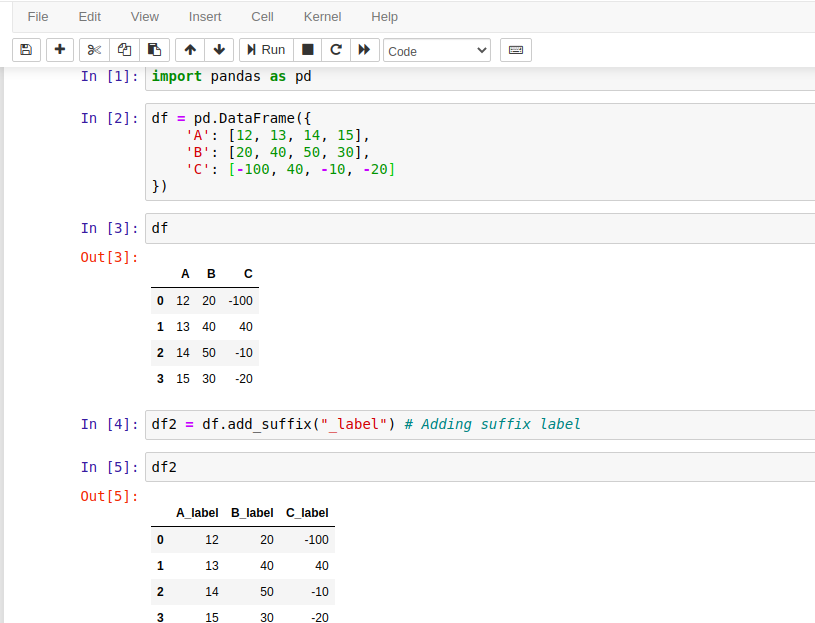# Python | Pandas DataFrame add_suffix() Method

Pandas add_suffix() method is a built-in method of DataFrame that is used to add a suffix to row label and columns label in Series and DataFrame respectively.

If we call this method on Series then it adds suffix label to row label similarly it adds suffix with columns label if called on DataFrame. Suffix value is a string value that is concatenated after with the previous label value.

For example, if a DataFrame has a column label as A then adding "Value" as a suffix will modify it as AValue. For more details see the examples below.

## Syntax

``DataFrame.add_suffix(suffix)``

### Parameters:

suffix: The string to add after each label.

### Return Value:

It returns either a new Series or new DataFrame with updated labels.

## Example with Jupyter Notebook

Let's take an example to understand the use of pandas.DataFrame.add_suffix() method. Following is the code that is executed by using the Jupyter notebook. If you are familiar with Jupyter, See the screenshot below.## Example: How to Set(suffix) Column Label to a DataFrame in Pandas?

We can use DataFrame.add_suffix() method to add string suffix for column labels in DataFrame. See the example here. This example is similar to the above code, executed by using Jupyter notebook. If you are not familiar with Jupyter then see this code to understand.

``````# import pandas library
import pandas as pd
# Create DataFrame of data
df = pd.DataFrame({
'A': [12, 13, 14, 15],
'B': [20, 40, 50, 30],
'C': [-100, 40, -10, 20]
})
# print dataframe
print(df)
print("\nDataFrame with updated labels...\n")
print(df2)
``````

Output:

A   B    C
0
12  20 -100
1  13  40   40
2  14  50  -10
3  15  30   20

DataFrame with updated labels...

A_label  B_label  C_label
0
12      20    -100
1      13      40      40
2      14      50     -10
3      15      30      20

## Example: Set(Suffix) Row Label To a Series in Pandas

If we are working with Series and want to modify its label by adding some suffix string then use the add_suffix() method. It returns a Series after modifying its labels. See the example here.

``````# import pandas library
import pandas as pd
# Create Series of data
sr = pd.Series([12, 13, 14, 15])
# print Series
print(sr)
print("\nSeries with updated labels...\n")
print(sr2)
``````

Output:

0    12
1    13
2    14
3    15
dtype: int64

Series with updated labels...

0_label    12
1_label    13
2_label    14
3_label    15
dtype: int64

## Example: Create DataFrame using Numpy in Pandas

Here, we have a DataFrame created by using the NumPy library and we are using the add_suffix() method to set column labels. See the example here.

``````# import pandas and numpy library
import pandas as pd
import numpy as np
# Creating DataFrame using numpy Array
df = pd.DataFrame(np.random.rand(5, 3), columns =['A', 'B', 'C'])
# Print DataFrame
print(df)
print("\nNew Dataframe after updating labels...\n")
# Print new dataframe
print(df2)
``````

Output:

A         B         C
0  0.267441  0.471324  0.876935
1  0.490577  0.622735  0.688777
2  0.171497  0.511526  0.813753
3  0.970845  0.249137  0.839091
4  0.667099  0.313956  0.486564

New Dataframe after updating labels...

A_RandomVal  B_RandomVal  C_RandomVal
0       0.267441       0.471324       0.876935
1       0.490577       0.622735       0.688777
2       0.171497       0.511526       0.813753
3       0.970845       0.249137       0.839091
4       0.667099       0.313956       0.486564

Trending